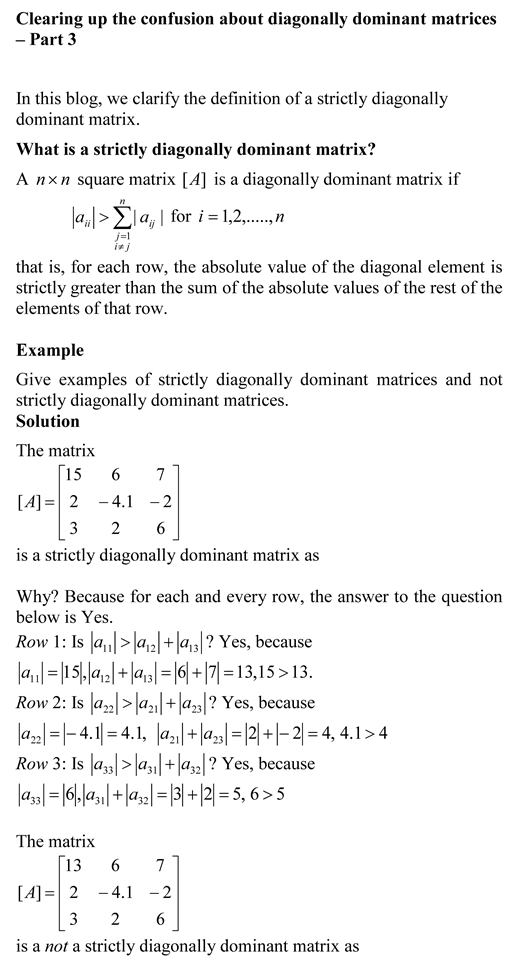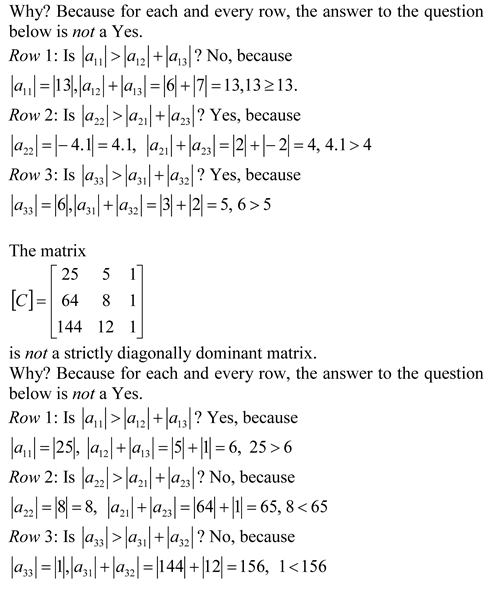## Matrix Algebra: Introduction

Many university STEM major programs have reduced the credit hours for a course in Matrix Algebra or have simply dropped the course from their curriculum.   The content of Matrix Algebra in many cases is taught just in time where needed.  This approach can leave a student with many conceptual holes in the required knowledge of matrix algebra.

In this series of blogs, we bring to you ten topics that are of immediate and intermediate interest for Matrix Algebra.

Here is the first topic where we define a matrix, vector, submatrix, square matrix, triangular matrix (upper and lower), diagonal matrix, identity matrix, and diagonally dominant matrix.  Get the information in form of textbook content, lecture videos, multiple choice test, problem set and PowerPoint presentation.

Introduction

This post is brought to you by

• Holistic Numerical Methods Open Course Ware:
• the textbooks on
• the Massive Open Online Course (MOOCs) available at

## Clearing up the confusion about diagonally dominant matrices – Part 3You can view the above document as a pdf file as well.

Other blogs on diagonally dominant matrices
Clearing up the confusion about diagonally dominant matrices – Part 1

Clearing up the confusion about diagonally dominant matrices – Part 2

_________________________________________________________

This post is brought to you by

• Holistic Numerical Methods Open Course Ware:
• the textbooks on
• the Massive Open Online Course (MOOCs) available at